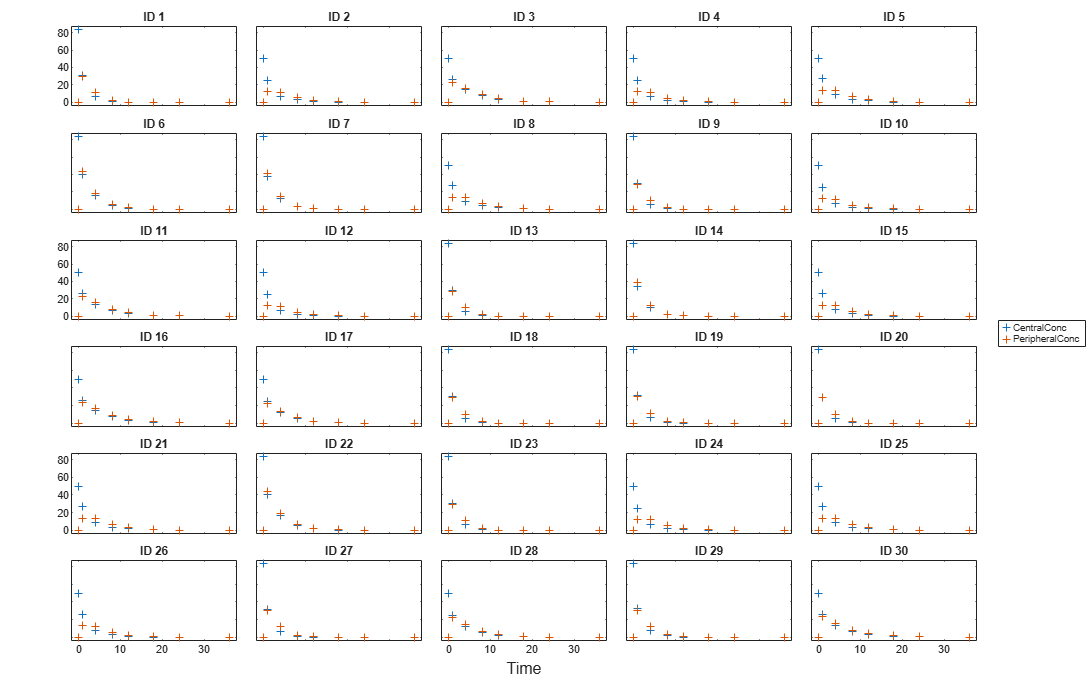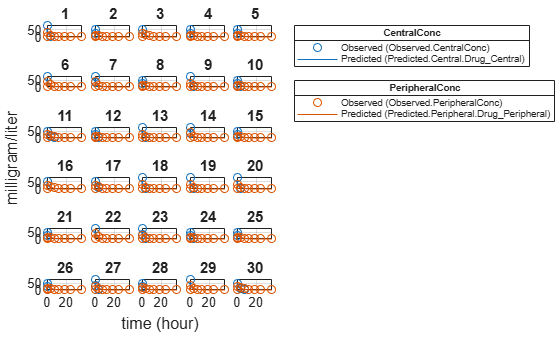# Estimate Category-Specific PK Parameters for Multiple Individuals

This example shows how to estimate category-specific (such as young versus old, male versus female), individual-specific, and population-wide parameters using PK profile data from multiple individuals.

### Background

Suppose you have drug plasma concentration data from 30 individuals and want to estimate pharmacokinetic parameters, namely the volumes of central and peripheral compartment, the clearance, and intercompartmental clearance. Assume the drug concentration versus the time profile follows the biexponential decline ${C}_{t}=A{e}^{-at}+B{e}^{-bt}$, where ${C}_{t}$ is the drug concentration at time t, and $a$ and $b$ are slopes for corresponding exponential declines.

This synthetic data contains the time course of plasma concentrations of 30 individuals after a bolus dose (100 mg) measured at different times for both central and peripheral compartments. It also contains categorical variables, namely `Sex` and `Age`.

```clear load('sd5_302RAgeSex.mat')```

### Convert to groupedData Format

Convert the data set to a `groupedData` object, which is the required data format for the fitting function `sbiofit`. A `groupedData` object also allows you set independent variable and group variable names (if they exist). Set the units of the `ID`, `Time`, `CentralConc`, `PeripheralConc`, `Age`, and `Sex` variables. The units are optional and only required for the `UnitConversion` feature, which automatically converts matching physical quantities to one consistent unit system.

```gData = groupedData(data); gData.Properties.VariableUnits = {'','hour','milligram/liter','milligram/liter','',''}; gData.Properties```
```ans = struct with fields: Description: '' UserData: [] DimensionNames: {'Row' 'Variables'} VariableNames: {1x6 cell} VariableDescriptions: {} VariableUnits: {1x6 cell} VariableContinuity: [] RowNames: {} CustomProperties: [1x1 matlab.tabular.CustomProperties] GroupVariableName: 'ID' IndependentVariableName: 'Time' ```

The `IndependentVariableName` and `GroupVariableName` properties have been automatically set to the `Time` and `ID` variables of the data.

### Visualize Data

Display the response data for each individual.

```t = sbiotrellis(gData,'ID','Time',{'CentralConc','PeripheralConc'},... 'Marker','+','LineStyle','none'); % Resize the figure. t.hFig.Position(:) = [100 100 1280 800];```### Set Up a Two-Compartment Model

Use the built-in PK library to construct a two-compartment model with infusion dosing and first-order elimination where the elimination rate depends on the clearance and volume of the central compartment. Use the `configset` object to turn on unit conversion.

```pkmd = PKModelDesign; pkc1 = addCompartment(pkmd,'Central'); pkc1.DosingType = 'Bolus'; pkc1.EliminationType = 'linear-clearance'; pkc1.HasResponseVariable = true; pkc2 = addCompartment(pkmd,'Peripheral'); model = construct(pkmd); configset = getconfigset(model); configset.CompileOptions.UnitConversion = true;```

For details on creating compartmental PK models using the SimBiology® built-in library, see Create Pharmacokinetic Models.

### Define Dosing

Assume every individual receives a bolus dose of 100 mg at time = 0. For details on setting up different dosing strategies, see Doses in SimBiology Models.

```dose = sbiodose('dose','TargetName','Drug_Central'); dose.StartTime = 0; dose.Amount = 100; dose.AmountUnits = 'milligram'; dose.TimeUnits = 'hour';```

### Map the Response Data to Corresponding Model Components

The data contains measured plasma concentration in the central and peripheral compartments. Map these variables to the appropriate model components, which are `Drug_Central` and `Drug_Peripheral`.

`responseMap = {'Drug_Central = CentralConc','Drug_Peripheral = PeripheralConc'};`

### Specify Parameters to Estimate

Specify the volumes of central and peripheral compartments `Central` and `Peripheral`, intercompartmental clearance `Q12`, and clearance `Cl_Central` as parameters to estimate. The `estimatedInfo` object lets you optionally specify parameter transforms, initial values, and parameter bounds. Since both `Central` and `Peripheral` are constrained to be positive, specify a log-transform for each parameter.

```paramsToEstimate = {'log(Central)', 'log(Peripheral)', 'Q12', 'Cl_Central'}; estimatedParam = estimatedInfo(paramsToEstimate,'InitialValue',[1 1 1 1]);```

### Estimate Individual-Specific Parameters

Estimate one set of parameters for each individual by setting the `'Pooled'` name-value pair argument to `false`.

`unpooledFit = sbiofit(model,gData,responseMap,estimatedParam,dose,'Pooled',false);`

### Display Results

Plot the fitted results versus the original data for each individual (group).

`plot(unpooledFit);`For an unpooled fit, `sbiofit` always returns one results object for each individual.

### Examine Parameter Estimates for Category Dependencies

Explore the unpooled estimates to see if there is any category-specific parameters, that is, if some parameters are related to one or more categories. If there are any category dependencies, it might be possible to reduce the number of degrees of freedom by estimating just category-specific values for those parameters.

First extract the ID and category values for each ID

`catParamValues = unique(gData(:,{'ID','Sex','Age'}));`

Add variables to the table containing each parameter's estimate.

```allParamValues = vertcat(unpooledFit.ParameterEstimates); catParamValues.Central = allParamValues.Estimate(strcmp(allParamValues.Name, 'Central')); catParamValues.Peripheral = allParamValues.Estimate(strcmp(allParamValues.Name, 'Peripheral')); catParamValues.Q12 = allParamValues.Estimate(strcmp(allParamValues.Name, 'Q12')); catParamValues.Cl_Central = allParamValues.Estimate(strcmp(allParamValues.Name, 'Cl_Central'));```

Plot estimates of each parameter for each category. `gscatter` requires Statistics and Machine Learning Toolbox™. If you do not have it, use other alternative plotting functions such as `plot`.

```h = figure; ylabels = ["Central","Peripheral","Q12","Cl\_Central"]; plotNumber = 1; for i = 1:4 thisParam = estimatedParam(i).Name; % Plot for Sex category subplot(4,2,plotNumber); plotNumber = plotNumber + 1; gscatter(double(catParamValues.Sex), catParamValues.(thisParam), catParamValues.Sex); ax = gca; ax.XTick = []; ylabel(ylabels(i)); legend('Location','bestoutside') % Plot for Age category subplot(4,2,plotNumber); plotNumber = plotNumber + 1; gscatter(double(catParamValues.Age), catParamValues.(thisParam), catParamValues.Age); ax = gca; ax.XTick = []; ylabel(ylabels(i)); legend('Location','bestoutside') end % Resize the figure. h.Position(:) = [100 100 1280 800];```Based on the plot, it seems that young individuals tend to have higher volumes of central and peripheral compartments (`Central`, `Peripheral`) than old individuals (that is, the volumes seem to be age-specific). In addition, males tend to have lower clearance rates (`Cl_Central`) than females but the opposite for the Q12 parameter (that is, the clearance and Q12 seem to be sex-specific).

### Estimate Category-Specific Parameters

Use the `'CategoryVariableName'` property of the `estimatedInfo` object to specify which category to use during fitting. Use `'Sex'` as the group to fit for the clearance `Cl_Central` and `Q12` parameters. Use `'Age'` as the group to fit for the `Central` and `Peripheral` parameters.

```estimatedParam(1).CategoryVariableName = 'Age'; estimatedParam(2).CategoryVariableName = 'Age'; estimatedParam(3).CategoryVariableName = 'Sex'; estimatedParam(4).CategoryVariableName = 'Sex'; categoryFit = sbiofit(model,gData,responseMap,estimatedParam,dose)```
```categoryFit = OptimResults with properties: ExitFlag: 3 Output: [1x1 struct] GroupName: [] Beta: [8x5 table] ParameterEstimates: [120x6 table] J: [240x8x2 double] COVB: [8x8 double] CovarianceMatrix: [8x8 double] R: [240x2 double] MSE: 0.4362 SSE: 205.8690 Weights: [] LogLikelihood: -477.9195 AIC: 971.8390 BIC: 1.0052e+03 DFE: 472 DependentFiles: {1x3 cell} EstimatedParameterNames: {'Central' 'Peripheral' 'Q12' 'Cl_Central'} ErrorModelInfo: [1x3 table] EstimationFunction: 'lsqnonlin' ```

When fitting by category (or group), `sbiofit` always returns one results object, not one for each category level. This is because both male and female individuals are considered to be part of the same optimization using the same error model and error parameters, similarly for the young and old individuals.

### Plot Results

Plot the category-specific estimated results.

`plot(categoryFit);`For the `Cl_Central` and `Q12` parameters, all males had the same estimates, and similarly for the females. For the `Central` and `Peripheral` parameters, all young individuals had the same estimates, and similarly for the old individuals.

### Estimate Population-Wide Parameters

To better compare the results, fit the model to all of the data pooled together, that is, estimate one set of parameters for all individuals by setting the `'Pooled'` name-value pair argument to `true`. The warning message tells you that this option will ignore any category-specific information (if they exist).

`pooledFit = sbiofit(model,gData,responseMap,estimatedParam,dose,'Pooled',true);`
```Warning: CategoryVariableName property of the estimatedInfo object is ignored when using the 'Pooled' option. ```

### Plot Results

Plot the fitted results versus the original data. Although a separate plot was generated for each individual, the data was fitted using the same set of parameters (that is, all individuals had the same fitted line).

`plot(pooledFit);`### Compare Residuals

Compare residuals of `CentralConc` and `PeripheralConc` responses for each fit.

```t = gData.Time; allResid(:,:,1) = pooledFit.R; allResid(:,:,2) = categoryFit.R; allResid(:,:,3) = vertcat(unpooledFit.R); h = figure; responseList = {'CentralConc', 'PeripheralConc'}; for i = 1:2 subplot(2,1,i); oneResid = squeeze(allResid(:,i,:)); plot(t,oneResid,'o'); refline(0,0); % A reference line representing a zero residual title(sprintf('Residuals (%s)', responseList{i})); xlabel('Time'); ylabel('Residuals'); legend({'Pooled','Category-Specific','Unpooled'}); end % Resize the figure. h.Position(:) = [100 100 1280 800];```As shown in the plot, the unpooled fit produced the best fit to the data as it fit the data to each individual. This was expected since it used the most number of degrees of freedom. The category-fit reduced the number of degrees of freedom by fitting the data to two categories (sex and age). As a result, the residuals were larger than the unpooled fit, but still smaller than the population-fit, which estimated just one set of parameters for all individuals. The category-fit might be a good compromise between the unpooled and pooled fitting provided that any hierarchical model exists within your data.